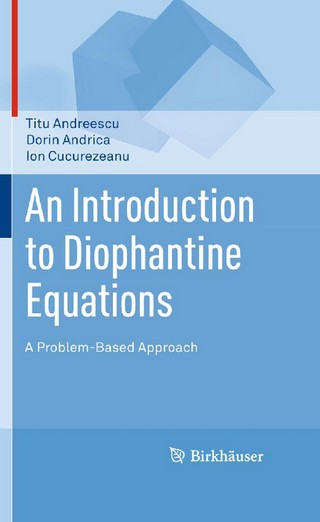### Book Toankho

Sách hay - Sách đẹp

# An Introduction to Diophantine Equations (A Problem-Based Approach)This problem-solving book is an introduction to the study of Diophantine equations, a class of equations in which only integer solutions are allowed. The presentation features some classical Diophantine equations, including linear, Pythagorean, and some higher degree equations, as well as exponential Diophantine equations. Many of the selected exercises and problems are original or are presented with original solutions. An Introduction to Diophantine Equations: A Problem-Based Approach is intended for undergraduates, advanced high school students and teachers, mathematical contest participants — including Olympiad and Putnam competitors — as well as readers interested in essential mathematics. The work uniquely presents unconventional and non-routine examples, ideas, and techniques.